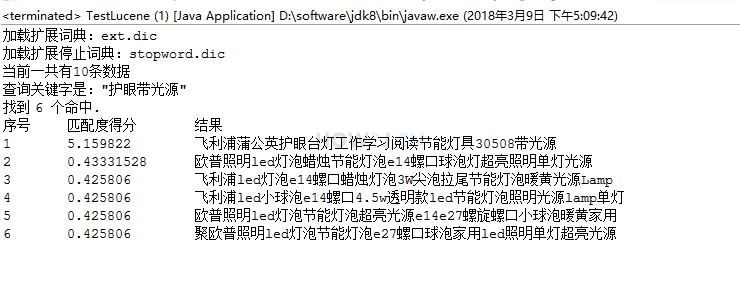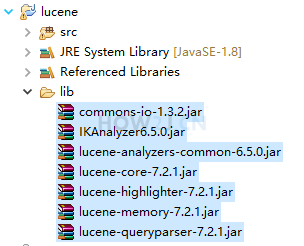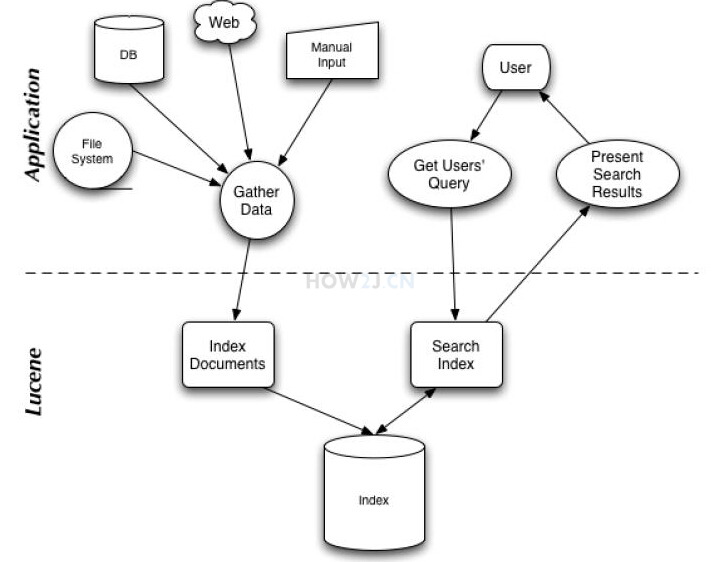# 搜索引擎技术Lucene7 入门

2022年4月18日 77点热度 0人点赞 0条评论

java零基础自学网站,点击了解:https://how2j.cn

### 步骤 3 : 先运行，看到效果，再学习### 步骤 6 : jar 包### 步骤 7 : TestLucene.java

 `package` `com.how2java;`   `import` `java.io.IOException;` `import` `java.io.StringReader;` `import` `java.util.ArrayList;` `import` `java.util.List;`   `import` `org.apache.lucene.analysis.TokenStream;` `import` `org.apache.lucene.document.Document;` `import` `org.apache.lucene.document.Field;` `import` `org.apache.lucene.document.TextField;` `import` `org.apache.lucene.index.DirectoryReader;` `import` `org.apache.lucene.index.IndexReader;` `import` `org.apache.lucene.index.IndexWriter;` `import` `org.apache.lucene.index.IndexWriterConfig;` `import` `org.apache.lucene.index.IndexableField;` `import` `org.apache.lucene.queryparser.classic.QueryParser;` `import` `org.apache.lucene.search.IndexSearcher;` `import` `org.apache.lucene.search.Query;` `import` `org.apache.lucene.search.ScoreDoc;` `import` `org.apache.lucene.search.highlight.Highlighter;` `import` `org.apache.lucene.search.highlight.QueryScorer;` `import` `org.apache.lucene.search.highlight.SimpleHTMLFormatter;` `import` `org.apache.lucene.store.Directory;` `import` `org.apache.lucene.store.RAMDirectory;` `import` `org.wltea.analyzer.lucene.IKAnalyzer;`   `public` `class` `TestLucene {`   `    ``public` `static` `void` `main(String[] args) ``throws` `Exception {` `        ``// 1. 准备中文分词器` `        ``IKAnalyzer analyzer = ``new` `IKAnalyzer();`   `        ``// 2. 索引` `        ``List productNames = ``new` `ArrayList<>();` `        ``productNames.add(``"飞利浦led灯泡e27螺口暖白球泡灯家用照明超亮节能灯泡转色温灯泡"``);` `        ``productNames.add(``"飞利浦led灯泡e14螺口蜡烛灯泡3W尖泡拉尾节能灯泡暖黄光源Lamp"``);` `        ``productNames.add(``"雷士照明 LED灯泡 e27大螺口节能灯3W球泡灯 Lamp led节能灯泡"``);` `        ``productNames.add(``"飞利浦 led灯泡 e27螺口家用3w暖白球泡灯节能灯5W灯泡LED单灯7w"``);` `        ``productNames.add(``"飞利浦led小球泡e14螺口4.5w透明款led节能灯泡照明光源lamp单灯"``);` `        ``productNames.add(``"飞利浦蒲公英护眼台灯工作学习阅读节能灯具30508带光源"``);` `        ``productNames.add(``"欧普照明led灯泡蜡烛节能灯泡e14螺口球泡灯超亮照明单灯光源"``);` `        ``productNames.add(``"欧普照明led灯泡节能灯泡超亮光源e14e27螺旋螺口小球泡暖黄家用"``);` `        ``productNames.add(``"聚欧普照明led灯泡节能灯泡e27螺口球泡家用led照明单灯超亮光源"``);    ` `        ``Directory index = createIndex(analyzer, productNames);`   `        ``// 3. 查询器` `        ``String keyword = ``"护眼带光源"``;` `        ``Query query = ``new` `QueryParser(``"name"``, analyzer).parse(keyword);` `        `  `        ``// 4. 搜索` `        ``IndexReader reader = DirectoryReader.open(index);` `        ``IndexSearcher searcher = ``new` `IndexSearcher(reader);` `        ``int` `numberPerPage = ``1000``;` `        ``System.out.printf(``"当前一共有%d条数据%n"``,productNames.size());` `        ``System.out.printf(``"查询关键字是："%s"%n"``,keyword);` `        ``ScoreDoc[] hits = searcher.search(query, numberPerPage).scoreDocs;`   `        ``// 5. 显示查询结果` `        ``showSearchResults(searcher, hits, query, analyzer);` `        ``// 6. 关闭查询` `        ``reader.close();` `    ``}`   `    ``private` `static` `void` `showSearchResults(IndexSearcher searcher, ScoreDoc[] hits, Query query, IKAnalyzer analyzer)` `            ``throws` `Exception {` `        ``System.out.println(``"找到 "` `+ hits.length + ``" 个命中."``);` `        ``System.out.println(``"序号t匹配度得分t结果"``);` `        ``for` `(``int` `i = ``0``; i < hits.length; ++i) {` `            ``ScoreDoc scoreDoc= hits[i];` `            ``int` `docId = scoreDoc.doc;` `            ``Document d = searcher.doc(docId);` `            ``List fields = d.getFields();` `            ``System.out.print((i + ``1``));` `            ``System.out.print(``"t"` `+ scoreDoc.score);` `            ``for` `(IndexableField f : fields) {` `                ``System.out.print(``"t"` `+ d.get(f.name()));` `            ``}` `            ``System.out.println();` `        ``}` `    ``}`   `    ``private` `static` `Directory createIndex(IKAnalyzer analyzer, List products) ``throws` `IOException {` `        ``Directory index = ``new` `RAMDirectory();` `        ``IndexWriterConfig config = ``new` `IndexWriterConfig(analyzer);` `        ``IndexWriter writer = ``new` `IndexWriter(index, config);`   `        ``for` `(String name : products) {` `            ``addDoc(writer, name);` `        ``}` `        ``writer.close();` `        ``return` `index;` `    ``}`   `    ``private` `static` `void` `addDoc(IndexWriter w, String name) ``throws` `IOException {` `        ``Document doc = ``new` `Document();` `        ``doc.add(``new` `TextField(``"name"``, name, Field.Store.YES));` `        ``w.addDocument(doc);` `    ``}` `}`

### 步骤 8 : 分词器

 `// 1. 准备中文分词器` `IKAnalyzer analyzer = new IKAnalyzer();`

### 步骤 9 : 创建索引

1. 首先准备10条数据

2. 通过createIndex方法，把它加入到索引当中

 `Directory index = ``new` `RAMDirectory();`

 `IndexWriterConfig config = ``new` `IndexWriterConfig(analyzer);`

 `IndexWriter writer = ``new` `IndexWriter(index, config);`

 `for` `(String name : products) {` `    ``addDoc(writer, name);` `}`

 `private` `static` `void` `addDoc(IndexWriter w, String name) ``throws` `IOException {` `    ``Document doc = ``new` `Document();` `    ``doc.add(``new` `TextField(``"name"``, name, Field.Store.YES));` `    ``w.addDocument(doc);` `}`
 `// 2. 索引` `List<``String``> productNames = new ArrayList<>();` `productNames.add("飞利浦led灯泡e27螺口暖白球泡灯家用照明超亮节能灯泡转色温灯泡");` `productNames.add("飞利浦led灯泡e14螺口蜡烛灯泡3W尖泡拉尾节能灯泡暖黄光源Lamp");` `productNames.add("雷士照明 LED灯泡 e27大螺口节能灯3W球泡灯 Lamp led节能灯泡");` `productNames.add("飞利浦 led灯泡 e27螺口家用3w暖白球泡灯节能灯5W灯泡LED单灯7w");` `productNames.add("飞利浦led小球泡e14螺口4.5w透明款led节能灯泡照明光源lamp单灯");` `productNames.add("飞利浦蒲公英护眼台灯工作学习阅读节能灯具30508带光源");` `productNames.add("欧普照明led灯泡蜡烛节能灯泡e14螺口球泡灯超亮照明单灯光源");` `productNames.add("欧普照明led灯泡节能灯泡超亮光源e14e27螺旋螺口小球泡暖黄家用");` `productNames.add("聚欧普照明led灯泡节能灯泡e27螺口球泡家用led照明单灯超亮光源");    ` `Directory index = createIndex(analyzer, productNames);`
 `private static Directory createIndex(IKAnalyzer analyzer, List<``String``> products) throws IOException {` `    ``Directory index = new RAMDirectory();` `    ``IndexWriterConfig config = new IndexWriterConfig(analyzer);` `    ``IndexWriter writer = new IndexWriter(index, config);`   `    ``for (String name : products) {` `        ``addDoc(writer, name);` `    ``}` `    ``writer.close();` `    ``return index;` `}`
 `private static void addDoc(IndexWriter w, String name) throws IOException {` `    ``Document doc = new Document();` `    ``doc.add(new TextField("name", name, Field.Store.YES));` `    ``w.addDocument(doc);` `}`

### 步骤 10 : 创建查询器

 `String keyword = "护眼带光源";` `Query query = new QueryParser("name", analyzer).parse(keyword);`

### 步骤 11 : 执行搜索

 `IndexReader reader = DirectoryReader.open(index);`

 `IndexSearcher searcher = ``new` `IndexSearcher(reader);`

 `int` `numberPerPage = ``1000``;`

 `ScoreDoc[] hits = searcher.search(query, numberPerPage).scoreDocs;`
 `// 4. 搜索` `IndexReader reader = DirectoryReader.open(index);` `IndexSearcher searcher = new IndexSearcher(reader);` `int numberPerPage = 1000;` `System.out.printf("当前一共有%d条数据%n",productNames.size());` `System.out.printf("查询关键字是："%s"%n",keyword);` `ScoreDoc[] hits = searcher.search(query, numberPerPage).scoreDocs;`

### 步骤 12 : 显示查询结果

 `for` `(``int` `i = ``0``; i < hits.length; ++i) {` `ScoreDoc scoreDoc= hits[i];`

 `int` `docId = scoreDoc.doc;`

 `Document d = searcher.doc(docId);`

scoreDoc.score 表示当前命中的匹配度得分，越高表示匹配程度越高

 `List fields = d.getFields();` `System.out.print((i + ``1``));` `System.out.print(``"t"` `+ scoreDoc.score);` `for` `(IndexableField f : fields) {` `        ``System.out.print(``"t"` `+ d.get(f.name()));` `}`
 `private static void showSearchResults(IndexSearcher searcher, ScoreDoc[] hits, Query query, IKAnalyzer analyzer)` `        ``throws Exception {` `    ``System.out.println("找到 " + hits.length + " 个命中.");` `    ``System.out.println("序号t匹配度得分t结果");` `    ``for (int i = 0; i < ``hits.length``; ++i) {` `        ``ScoreDoc ``scoreDoc``= ``hits``[i];` `        ``int ``docId` `= ``scoreDoc``.doc;` `        ``Document ``d` `= ``searcher``.doc(docId);` `        ``List fields = d.getFields();` `        ``System.out.print((i + 1));` `        ``System.out.print("t" + scoreDoc.score);` `        ``for (IndexableField f : fields) {` `            ``System.out.print("t" + d.get(f.name()));` `        ``}` `        ``System.out.println();` `    ``}` `}`

### 步骤 13 : 运行结果### 步骤 14 : 和 like 的区别

like 也可以进行查询，那么使用lucene 的方式有什么区别呢？ 主要是两点：
1. 相关度

2. 性能

### 步骤 15 : 思路图

1. 首先搜集数据

2. 通过数据创建索引
3. 用户输入关键字
4. 通过关键字创建查询器
5. 根据查询器到索引里获取数据
6. 然后把查询结果展示在用户面前zdc1228@163.com